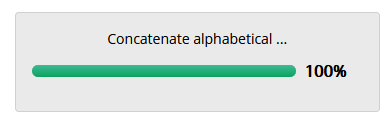## Problem Statement :

Given a non-empty string instr containing only alphabets. Print the sting outstr based on the below logic:

• Form alphabetical groups from the alphabets in instr

An alphabetical group will contain all the occurrences of an alphabet, both in lower case and upper case, in their order of occurrences in instr

• Arrange all the alphabetical groups formed in order from group A to group Z

• Concatenate the arranged alphabetical groups to outstr in the order given below:

First group, last group, second group, second last group and so on

Input format:

Read the string instr from the standard input stream.

Output format:

Print outstr to the standard output stream.## Question :## Code Solution :

```				```
x=input()
list1=[]
dick={}
s=""
for i in x:
if i.lower() not in dick:
dick[i.lower()]=i
else:
dick[i.lower()]+=i
for i in dick.values():
list1.append(i)
for i in range(len(list1)):
for j in range(i+1,len(list1)):
if list1[i].lower()>list1[j].lower():
list1[i],list1[j]=list1[j],list1[i]
for i in range(len(list1)//2):
s+=list1[i]+list1[len(list1)-1-i]
if len(list1)%2!=0:
s+=list1[len(list1)//2]
print(s)
```
```